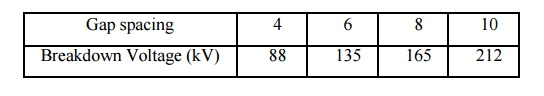Home | | High Voltage Engineering | Solved Problems: Over(High) Voltages in Electrical Power Systems

# Solved Problems: Over(High) Voltages in Electrical Power Systems

High Voltage Engineering - Over Voltages in Electrical Power Systems - Solved Problems: Over(High) Voltages in Electrical Power Systems

PROBLEMS

1.  A steady current of 600 ÎĽA flows through the plane electrode separated by a distance of 0.5 cm when a voltage of 10 kV is applied. Determine the Townsendâ€™s first ionization coefficient if a current of 60 ÎĽA flows when the distance of separation is reduced to 0.1 cm and the field is kept constant at the previous value.

Solution: Since the field is kept constant (i.e., if distance of separation is reduced, the voltage is also reduced by the same ratio so that V/d is kept constant).

I = I0 eÎ±x

Substituting two different sets of values,

we have 600 = I0 e0.5Î± and 60 = I0 e0.1Î±

or 10 = e0.4Î±  or 0.4 Î± = 1n 10

0.4Î± = 2.3026

Î±= 5.75 ionizing collisions/cm.

2.  The following table gives two sets of experimental results for studying Townsendâ€™s mechanism. The field is kept constant in each set:The maximum current observed is 6 Ă— 10â€“14 A. Determine the values of Townsendâ€™s first and second ionization coefficients.

3. The following observations were made in an experiment for determination of dielectric strength of transformer oil. Determine the power law equation.Study Material, Lecturing Notes, Assignment, Reference, Wiki description explanation, brief detail
High Voltage Engineering : Electrical breakdown is gases, solids& Liquids : Solved Problems: Over(High) Voltages in Electrical Power Systems |